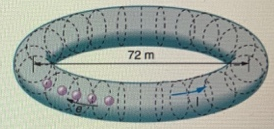# Problem: The Stanford Linear Accelerator Center (SLAC) operated an electron storage ring about 72.0 m in diameter called SPEAR (closed in 2009), in which a circulating beam of electrons moved at nearly the speed of light. Assuming that this electron beam had a current of 17 A, how many electrons were in the beam at any given moment?

###### FREE Expert Solution

Charge:

$\overline{){\mathbf{Q}}{\mathbf{=}}{\mathbf{n}}{{\mathbf{q}}}_{{\mathbf{e}}}}$

n is the number of particles and qe is the charge of an electron.

Current:

$\overline{){\mathbf{i}}{\mathbf{=}}\frac{\mathbf{∆}\mathbf{Q}}{\mathbf{∆}\mathbf{t}}}$

Speed, distance and time relationship:

$\overline{){\mathbf{v}}{\mathbf{=}}\frac{\mathbf{d}}{\mathbf{t}}}$

t = d/v = (πD)/v

Where d is the distance covered going round the ring and D is the diameter of the ring.

98% (187 ratings)###### Problem Details

The Stanford Linear Accelerator Center (SLAC) operated an electron storage ring about 72.0 m in diameter called SPEAR (closed in 2009), in which a circulating beam of electrons moved at nearly the speed of light.Assuming that this electron beam had a current of 17 A, how many electrons were in the beam at any given moment?